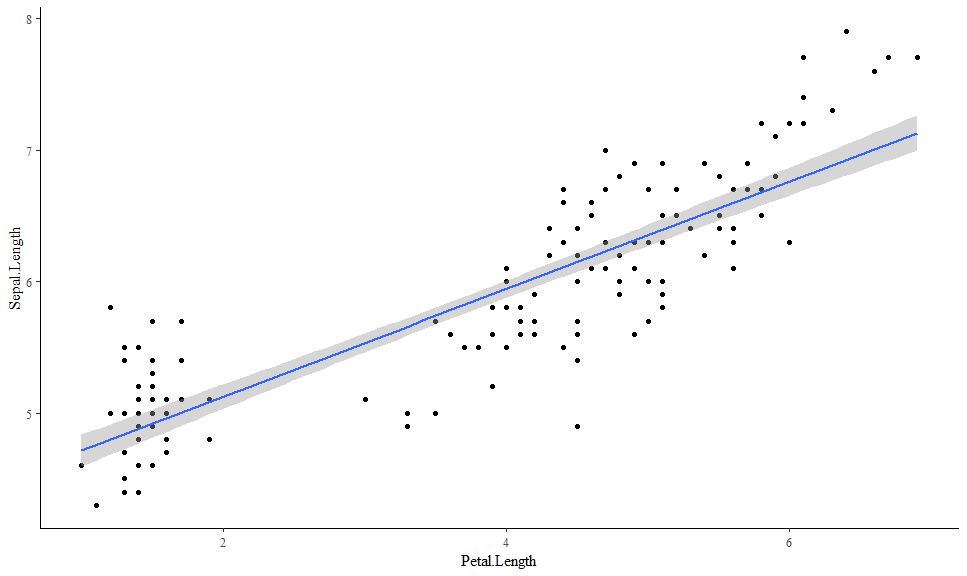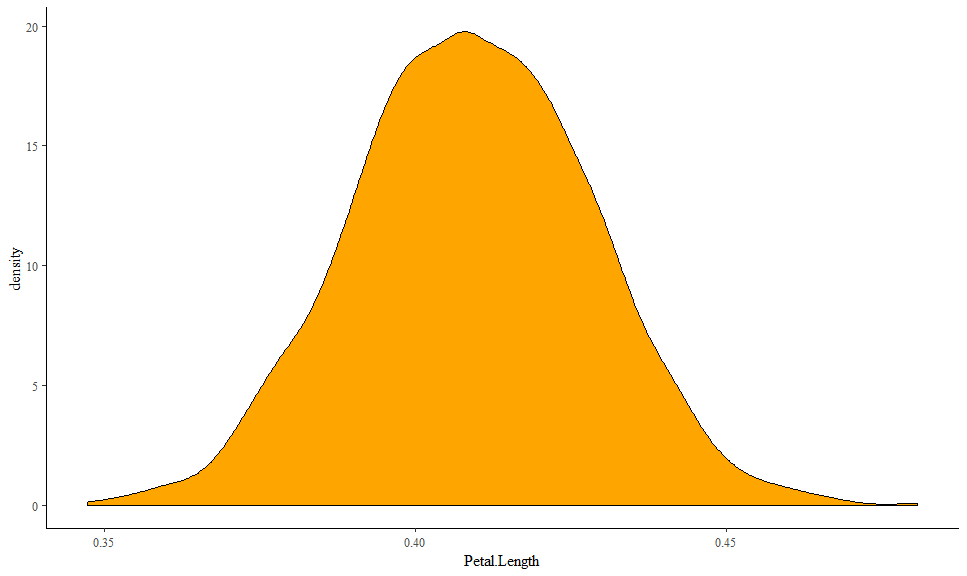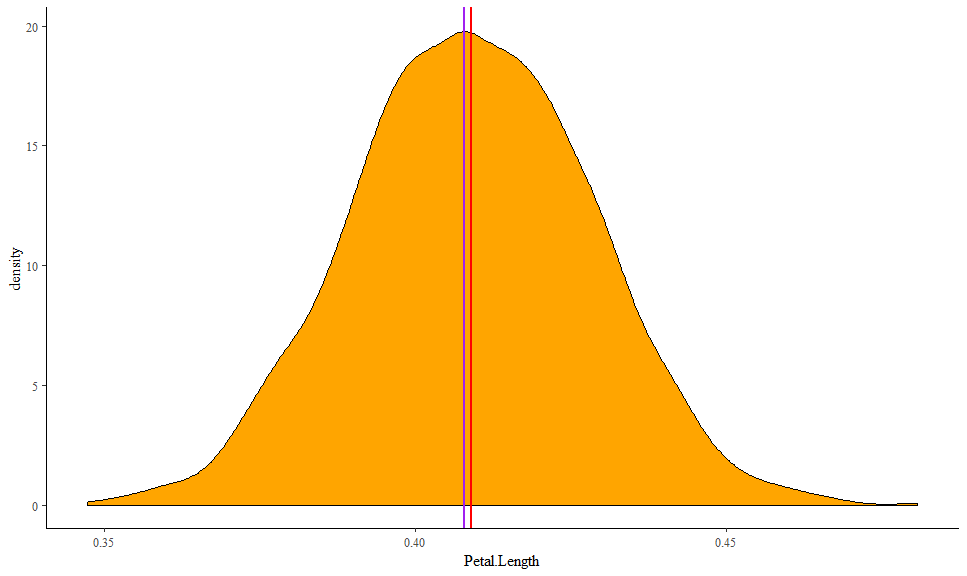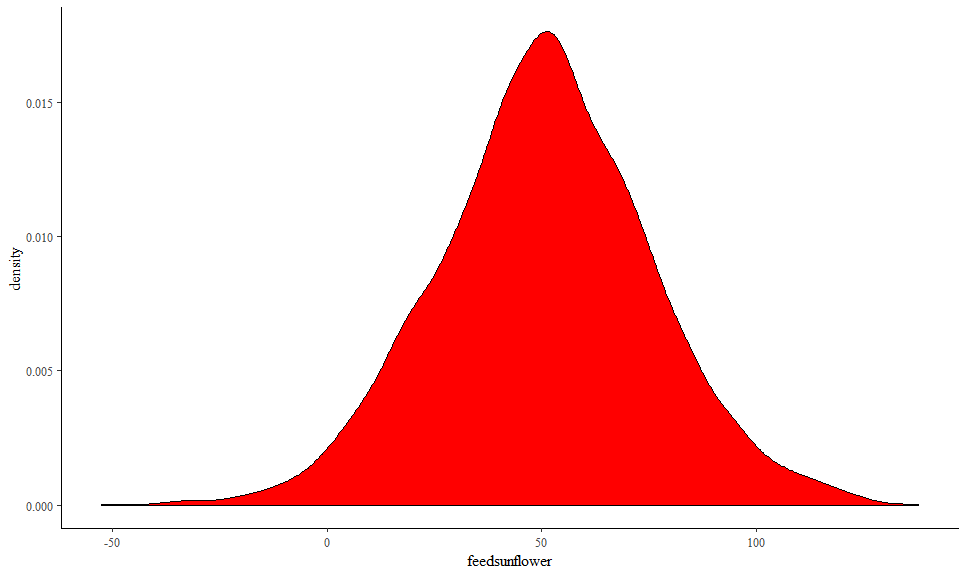# Example 1: Initiation to Bayesian models

This vignette can be referred to by citing the package:

Now that you’ve read the Get started section, let’s dive in the subtleties of Bayesian modelling using R.

Once you’ve installed the necessary packages, we can load `rstanarm` (to fit the models), `bayestestR` (to compute useful indices) and `insight` (to access the parameters).

``````library(rstanarm)
library(bayestestR)
library(insight)``````

## Simple linear model (aka a regression)

We will begin by a simple regression to test the relationship between Petal.Length (our predictor, - or independent, variable) and Sepal.Length (our response, - or dependent, variable) from the `iris` dataset, included by default in R.

### Fitting the model

Let’s start by fitting the frequentist version of the model, just to have some reference:

``````model <- lm(Sepal.Length ~ Petal.Length, data=iris)
summary(model)``````
``````>
> Call:
> lm(formula = Sepal.Length ~ Petal.Length, data = iris)
>
> Residuals:
>     Min      1Q  Median      3Q     Max
> -1.2468 -0.2966 -0.0152  0.2768  1.0027
>
> Coefficients:
>              Estimate Std. Error t value Pr(>|t|)
> (Intercept)    4.3066     0.0784    54.9   <2e-16 ***
> Petal.Length   0.4089     0.0189    21.6   <2e-16 ***
> ---
> Signif. codes:  0 '***' 0.001 '**' 0.01 '*' 0.05 '.' 0.1 ' ' 1
>
> Residual standard error: 0.41 on 148 degrees of freedom
> Multiple R-squared:  0.76,    Adjusted R-squared:  0.758
> F-statistic:  469 on 1 and 148 DF,  p-value: <2e-16
``````

In this model, the linear relationship between Petal.Length and Sepal.Length is positive and significant (beta = 0.41, t(148) = 21.6, p < .001). This means that for every increase of 1 in Petal.Length (the predictor), Sepal.Length (the response) increases by 0.41. This can be visualized by plotting, using the `ggplot2` package, the predictor values on the `x` axis and the response values as `y`:

``````library(ggplot2)  # Load the package

# The ggplot function takes the data as argument, and then the variables
# related to aesthetic features such as the x and y axes.
ggplot(iris, aes(x=Petal.Length, y=Sepal.Length)) +
geom_point() +  # This adds the points
geom_smooth(method="lm") # This adds a regression line``````Let’s fit a Bayesian version of the model:

``model <- stan_glm(Sepal.Length ~ Petal.Length, data=iris)``

You can see the sampling algorithm being run.

### Extracting the posterior

Once it is done, let us extract the parameters (i.e., the coefficients) of the model.

``````posteriors <- insight::get_parameters(model)

head(posteriors)  # Show the first 6 rows``````
``````>   (Intercept) Petal.Length
> 1         4.4         0.40
> 2         4.5         0.37
> 3         4.3         0.41
> 4         4.4         0.40
> 5         4.3         0.41
> 6         4.3         0.42
``````

As we can see, the parameters take the form of a long dataframe with two columns, corresponding to the intercept and the effect of Petal.Length. These columns contain the posterior distributions of these two parameters, i.e., a set of different plausible values.

Let’s look at the length of the posteriors.

``nrow(posteriors)  # Size (number of rows)``
``````>  4000
``````

Why is the size 4000, and not more or less?

First of all, these observations (the rows) are usually referred to as posterior draws. The underlying idea is that the Bayesian sampling algorithm (such as Monte Carlo Markov Chains - MCMC) will draw from the hidden true posterior distribution. Thus, it is through these posterior draws that we can estimate the underlying true posterior distribution. The more draws you have, the better is your estimation of the posterior distribution. But it also takes longer to compute.

If we look at the documentation (`?sampling`) for the rstanarm `"sampling"` algorithm used by default in the model above, we can see several parameters that influence the number of posterior draws. By default, there are 4 `chains` (you can see it as distinct sampling runs), that each create 2000 `iter` (draws). However, only half of these iterations is kept, as half is used for `warm-up` (the convergence of the algorithm). The total is `4 chains * (2000 iterations - 1000 warm-up) = 4000` posterior draws. We can change that, for instance:

``````model <- stan_glm(Sepal.Length ~ Petal.Length, data=iris, chains = 2, iter = 1000, warmup = 250)

nrow(insight::get_parameters(model))  # Size (number of rows)``````
`````` 1500
``````

In this case, we indeed have `2 chains * (1000 iterations - 250 warm-up) = 1500` posterior draws. Let’s keep our first model with the default setup.

#### Visualizing the posterior distribution

Now that we’ve understood where do these values come from, let’s look at them. We will start by visualizing the distribution of our parameter of interest, the effect of `Petal.Length`.

``````ggplot(posteriors, aes(x = Petal.Length)) +
geom_density(fill = "orange")``````This distribution represents the probability (the y axis) of different effects (the x axis). The central values are more probable than the extreme values. As you can see, this distribution ranges from about 0.35 to 0.50, with the bulk of it being at around 0.41.

Congrats! You’ve just described your posterior distribution.

And this is at the heart of Bayesian analysis. We don’t need p-values, t-values or degrees of freedom: everything is there, under our nose, within this posterior distribution.

As you can see, our description is consistent with the values of the frequentist regression (which had a beta of 0.41). This is reassuring! And indeed, in most cases, a Bayesian analysis does not drastically change the results or their interpretation. Rather, it makes the results more interpretable, intuitive and easy to understand and describe.

We can now go ahead and precisely characterize this posterior distribution.

### Describing the Posterior

Unfortunately, it is often not practical to report the whole posterior distributions as graphs. We need to find a concise way to summarize it. We recommend to describe the posterior distribution with 3 elements. A point-estimate, a one-value summary (similar to the beta in frequentist regressions); a credible interval, representing the associated uncertainty and some indices of significance, giving information about the importance of this effect.

#### Point-estimate

What single value can best represent my posterior distribution?

Centrality indices, such as the mean, the median or the mode are usually used as point-estimates. What’s the difference? Let’s check the mean:

``mean(posteriors\$Petal.Length)``
``````>  0.41
``````

This is close to the frequentist beta. But as we know, the mean is quite sensitive to outliers or extremes values. Maybe the median could be more robust?

``median(posteriors\$Petal.Length)``
``````>  0.41
``````

Well, this is close to the mean. Maybe we could take the mode, the peak of the posterior distribution? In the Bayesian framework, this value is called the Maximum A Posteriori (MAP). Let’s see:

``map_estimate(posteriors\$Petal.Length)``
``````> MAP = 0.41
``````

They are all very close! Let’s visualize these values on the posterior distribution:

``````ggplot(posteriors, aes(x = Petal.Length)) +
geom_density(fill = "orange") +
# The mean in blue
geom_vline(xintercept=mean(posteriors\$Petal.Length), color="blue", size=1) +
# The median in red
geom_vline(xintercept=median(posteriors\$Petal.Length), color="red", size=1) +
# The MAP in purple
geom_vline(xintercept=map_estimate(posteriors\$Petal.Length), color="purple", size=1)``````Well, all these values give very similar results. Thus, we will chose the median, as this value has a direct meaning from a probabilistic perspective: there is 50% chance that the true effect is higher and 50% chance that the effect is lower (as it cuts the distribution in two equal parts).

#### Uncertainty

Now that the have a point-estimate, we have to describe the uncertainty. We could compute the range:

``range(posteriors\$Petal.Length)``
``````>  0.35 0.48
``````

But does it make sense to include all these extreme values? Probably not. Thus, we will compute a credible interval. Long story short, it’s kind of similar to a frequentist confidence interval, but it is easier to interpret and easier to compute. And it makes more sense.

We will compute this credible interval based on the Highest Density Interval (HDI). It will give us the range containing the 89% more probable effect values. Note that we will use 89% CIs instead of 95% CIs (as in the frequentist framework), as the 89% level gives more stable results (Kruschke 2014) and reminds us about the arbitrarity of such conventions (McElreath 2018).

``hdi(posteriors\$Petal.Length, ci=0.89)``
``````> # Highest Density Interval
>
>       89% HDI
>  [0.38, 0.44]
``````

Nice, so we can conclude that the effect has 89% chance of falling within the `[0.38, 0.44]` range. We have just computed the two most important information to describe our effects.

#### Effect significance

However, in many scientific fields, simply describing the effect is not enough. The readers also want to know if this effect means something. Is it important? Is it different from 0? In other words, assessing the significance of the effect. How can we do this?

Well, in this particular case, it is very eloquent: all possible effect values (i.e., the whole posterior distribution) are positive and superior to 0.35, which is already a lot.

But still, we want some objective decision criterion, to say if yes or no the effect is significant. We could do similarly to the frequentist framework, and see if the Credible Interval contains 0. Here, it is not the case, which means that our effect is significant.

But this index is not very fine-grained, isn’t it? Can we do better? Yes.

## A linear model with a categorical predictor

Let’s take interest in the weight of chickens, depending on 2 feed types. We will start by selecting, from the `chickwts` dataset (also available in base R), the 2 feed types that are of interest for us (because reasons): Meat meals and Sunflowers.

### Data preparation and model fitting

``````library(dplyr)

# We keep only rows for which feed is meatmeal or sunflower
data <- chickwts %>%
filter(feed %in% c("meatmeal", "sunflower"))``````

Let’s run another Bayesian regression to predict the Weight with the 2 types of feed type.

``model <- stan_glm(weight ~ feed, data=data)``

### Posterior description

``````posteriors <- insight::get_parameters(model)

ggplot(posteriors, aes(x=feedsunflower)) +
geom_density(fill = "red")``````This represents the posterior distribution of the difference between meat meals and Sunflowers. Seems that the difference is rather positive… Eating Sunflowers makes you more fat (if you’re a chicken). By how much? Let us compute the median and the CI:

``median(posteriors\$feedsunflower)``
``````>  51
``````
``hdi(posteriors\$feedsunflower)``
``````> # Highest Density Interval
>
>        89% HDI
>  [7.77, 87.66]
``````

It makes you fat by around `51` (the median). However, the uncertainty is quite high: there is 89% chance that the difference between the two feed types is between `7.77` and `87.66`.

Is this effect different from 0?

### ROPE Percentage

Testing whether this distribution is different from 0 doesn’t make sense, as 0 is a single value (and the probability that any distribution is different from a single value is infinite).

However, one way to assess significance could be to define an area around 0, which will consider as equivalent to zero (to no, - or negligible, effect). This is called the Region of Practical Equivalence (ROPE), and is one way of testing the significance of parameters.

How can we define this region?

Driing driiiing

The easystats team speaking. How can we help?

I am Prof Howard. An expert in chicks… I mean chickens. Just calling to let you know that based on my expert knowledge, an effect between -20 and 20 is negligible. Bye.

Well, that’s convenient. Now we know that we can define the ROPE as the `[-20, 20]` range. All effects within this range are considered as null (negligible). We can now compute the proportion of the 89% most probable values (the 89% CI) which are not null, i.e., which are outside this range.

``rope(posteriors\$feedsunflower, range = c(-20, 20), ci=0.89)``
``````> # Proportion of samples inside the ROPE [-20.00, 20.00]:
>
>  inside ROPE
>       7.75 %
``````

7.75% of the 89% CI can be considered as null. Is that a lot? Based on our guidelines, yes, it is too much. Based on this particular definition of ROPE, we conclude that this effect is not significant (the probability of being negligible is too high).

Although, to be honest, I have some doubts about this Prof Howard. I don’t really trust his definition of ROPE. Is there a more objective way of defining it?

Yes. One of the practice is for instance to use the tenth (`1/10 = 0.1`) of the standard deviation (SD) of the response variable, which can be considered as a “negligible” effect size.

``````rope_value <- 0.1 * sd(data\$weight)
rope_range <- c(-rope_value, rope_value)
rope_range``````
``````>  -6.2  6.2
``````

Let’s redefine our ROPE as the region within the `[-6.2, 6.2]` range. Note that this can be directly obtained by the `rope_range` function :)

``````rope_value <- rope_range(model)
rope_range``````
``````>  -6.2  6.2
``````

Let’s recompute the percentage in ROPE:

``rope(posteriors\$feedsunflower, range = rope_range, ci=0.89)``
``````> # Proportion of samples inside the ROPE [-6.17, 6.17]:
>
>  inside ROPE
>       0.00 %
``````

With this reasonable definition of ROPE, we observe that the 89% of the posterior distribution of the effect does not overlap with the ROPE. Thus, we can conclude that the effect is significant (in the sense of important enough to be noted).

### Probability of Direction (pd)

Maybe we are not interested in whether the effect is not-negligible. Maybe we just want to know if this effect is positive or negative. In this case, we can simply compute the proportion of the posterior that is positive, no matter the “size” of the effect.

``````n_positive <- posteriors %>%
filter(feedsunflower > 0) %>% # select only positive values
nrow() # Get length
n_positive / nrow(posteriors) * 100``````
``````>  "97.82"
``````

We can conclude that the effect is positive with a probability of 97.82%. We call this index the Probability of Direction (pd). It can, in fact, be computed more easily with the following:

``p_direction(posteriors\$feedsunflower)``
``````> # Probability of Direction (pd)
>
> pd = 97.82%
``````

Interestingly, it so happens that this index is usually highly correlated with the frequentist p-value. We could almost roughly infer the corresponding p-value with a simple transformation:

``````pd <- 97.82
onesided_p <- 1 - pd / 100
twosided_p <- onesided_p * 2
twosided_p``````
``````>  0.044
``````

If we ran our model in the frequentist framework, we should approximately observe an effect with a p-value of 0.04. Is that true?

### Comparison to frequentist

``````lm(weight ~ feed, data=data) %>%
summary()``````
``````>
> Call:
> lm(formula = weight ~ feed, data = data)
>
> Residuals:
>     Min      1Q  Median      3Q     Max
> -123.91  -25.91   -6.92   32.09  103.09
>
> Coefficients:
>               Estimate Std. Error t value Pr(>|t|)
> (Intercept)      276.9       17.2   16.10  2.7e-13 ***
> feedsunflower     52.0       23.8    2.18     0.04 *
> ---
> Signif. codes:  0 '***' 0.001 '**' 0.01 '*' 0.05 '.' 0.1 ' ' 1
>
> Residual standard error: 57 on 21 degrees of freedom
> Multiple R-squared:  0.185,   Adjusted R-squared:  0.146
> F-statistic: 4.77 on 1 and 21 DF,  p-value: 0.0405
``````

The frequentist model tells us that the difference is positive and significant (beta = 52, p = 0.04).

Although we arrived to a similar conclusion, the Bayesian framework allowed us to develop a more profound and intuitive understanding of our effect, and of the uncertainty of its estimation.

## All with one function

And yet, I agree, it was a bit tedious to extract and compute all the indices. But what if I told you that we can do all of this, and more, with only one function?

Behold, `describe_posterior`!

This function computes all of the adored mentioned indices, and can be run directly on the model:

``describe_posterior(model)``
``````>       Parameter Median CI CI_low CI_high  pd ROPE_CI ROPE_low ROPE_high
> 1   (Intercept)    277 89  250.2     307 100      89     -6.2       6.2
> 2 feedsunflower     51 89    7.8      88  98      89     -6.2       6.2
>   ROPE_Percentage  ESS Rhat Prior_Distribution Prior_Location Prior_Scale
> 1               0 3437    1             normal              0         617
> 2               0 3316    1             normal              0         154
``````

Tada! There we have it! The median, the CI, the pd and the ROPE percentage!

Understanding and describing posterior distributions is just one aspect of Bayesian modelling… Are you ready for more? Click here to see the next example.

Kruschke, John. 2014. Doing Bayesian Data Analysis: A Tutorial with R, Jags, and Stan. Academic Press.

McElreath, Richard. 2018. Statistical Rethinking: A Bayesian Course with Examples in R and Stan. Chapman; Hall/CRC.